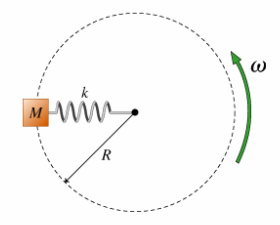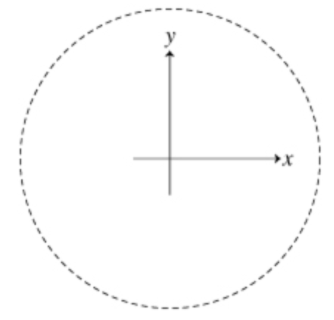# Problem: An object of mass M is attached to a spring with spring constant k whose unstretched length is L, and whose far end is fixed to a shaft that is rotating with angular speed ω. Neglect gravity and assume that the mass rotates with angular speed omega as shown. (Figure 1) When solving this problem use an inertial coordinate system, as drawn here. (Figure 2) What is happening to the spring as the angular velocity approaches ωcrit? A. The spring stretches linearly then breaks at ω = ωcrit. B. The value of ωcrit is so large that the spring will behave linearly for any practically attainable ω. C. As ωc approaches ωcrit the spring stops behaving linearly and begins to act more like an unstretchable rod until it eventually breaks.

###### FREE Expert Solution

In this problem, we are required to explain the behaviour of springs.

A spring extends linearly until it reaches its elastic limit.

89% (484 ratings)###### Problem Details

An object of mass M is attached to a spring with spring constant k whose unstretched length is L, and whose far end is fixed to a shaft that is rotating with angular speed ω. Neglect gravity and assume that the mass rotates with angular speed omega as shown. (Figure 1) When solving this problem use an inertial coordinate system, as drawn here. (Figure 2)What is happening to the spring as the angular velocity approaches ωcrit

A. The spring stretches linearly then breaks at ω = ωcrit

B. The value of ωcrit is so large that the spring will behave linearly for any practically attainable ω.

C. As ωc approaches ωcrit the spring stops behaving linearly and begins to act more like an unstretchable rod until it eventually breaks.